# Concrete Footing Calculator

Calculate the concrete material needed to pour various types of footings, including pads, foundations, and piers. Get the results in cubic yards or bags.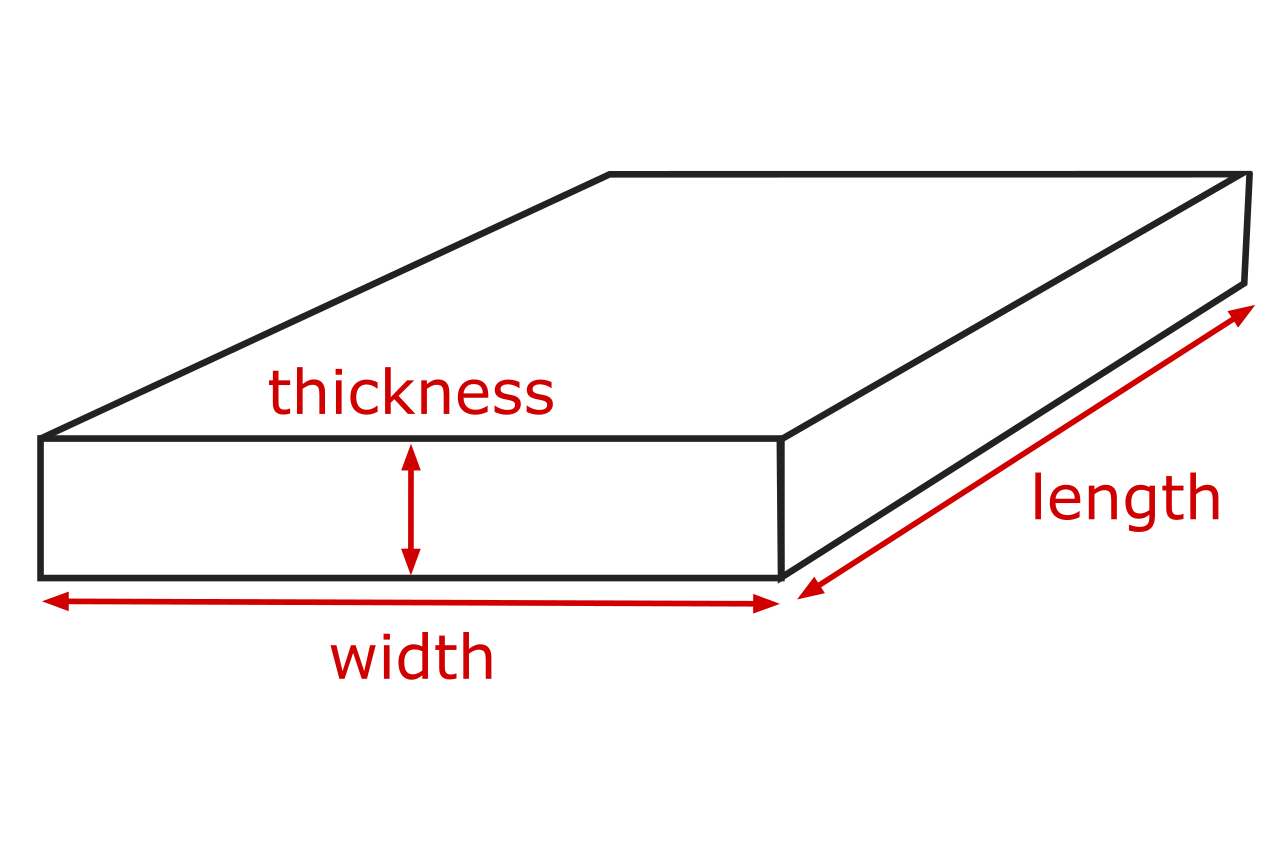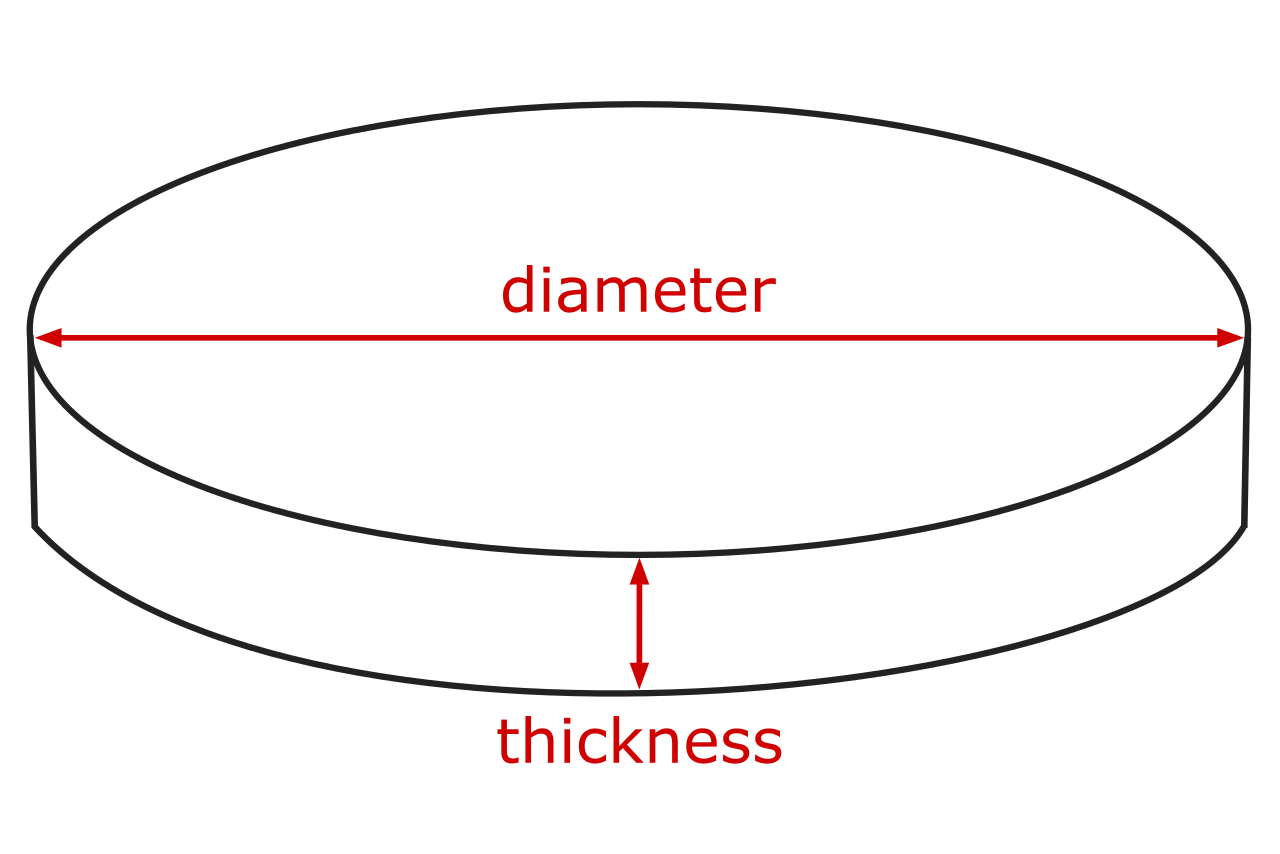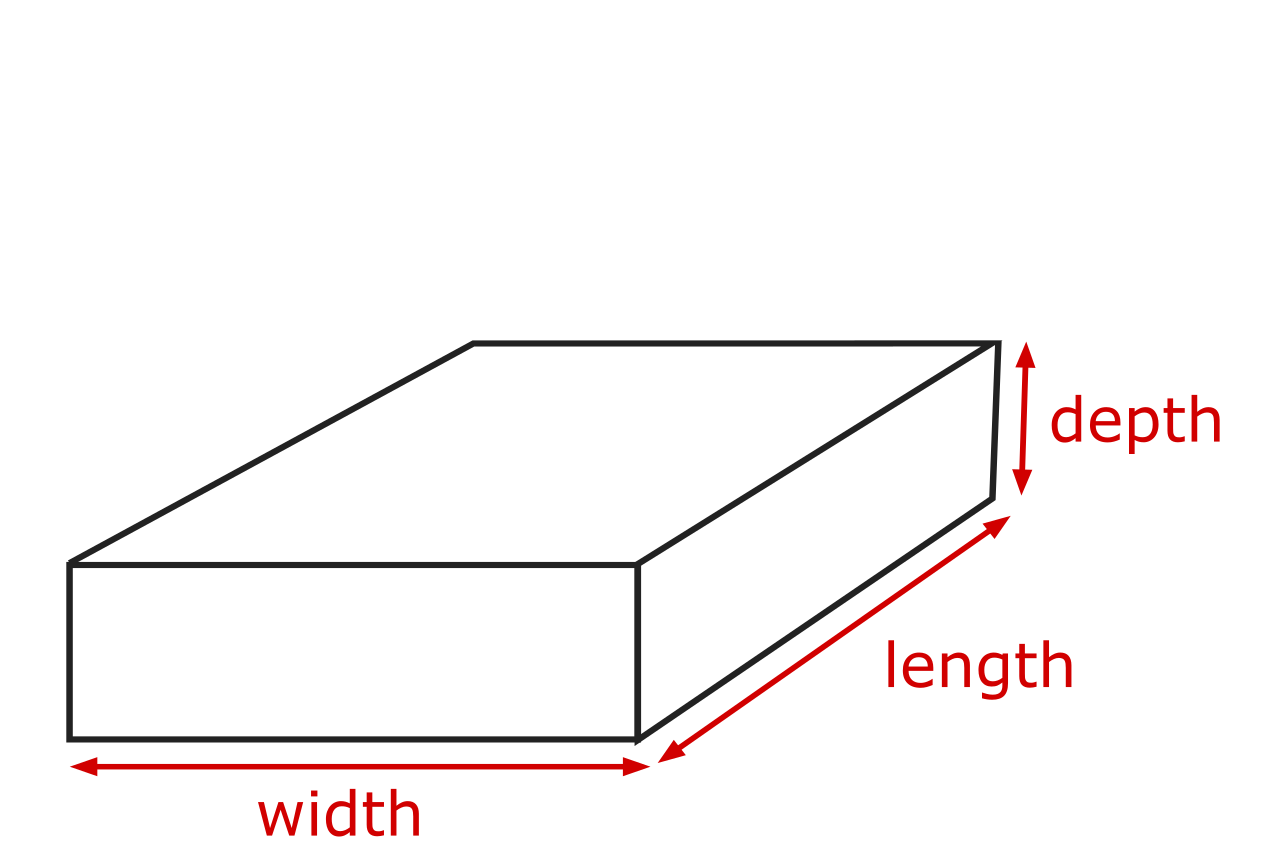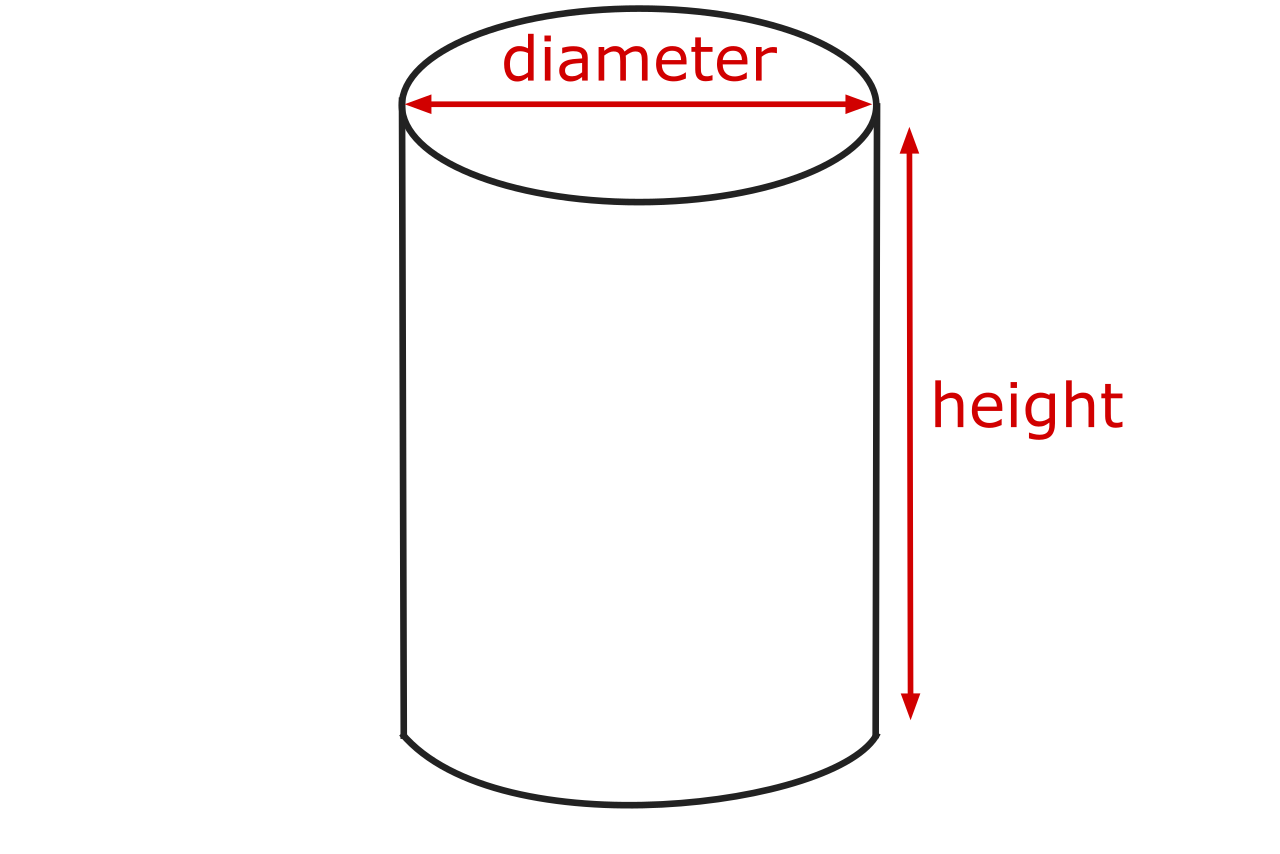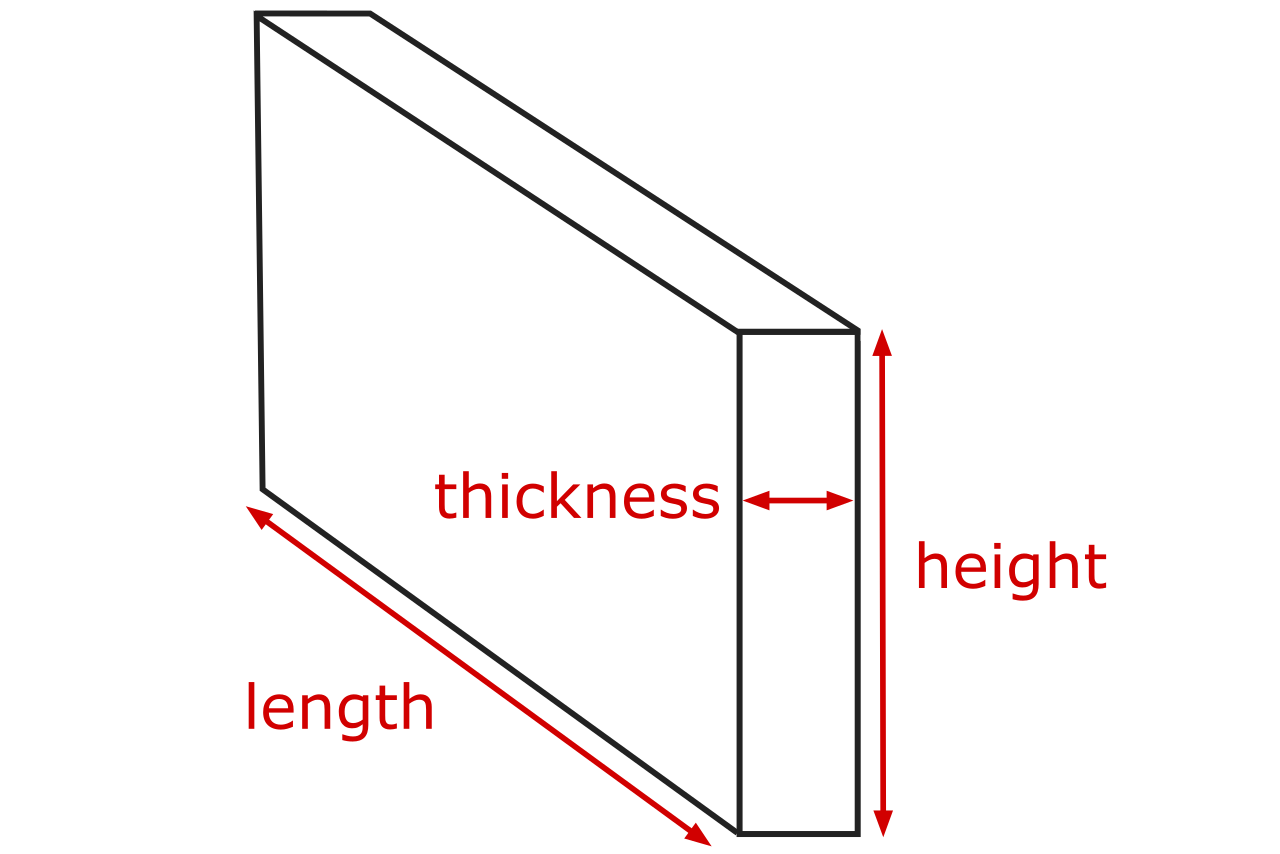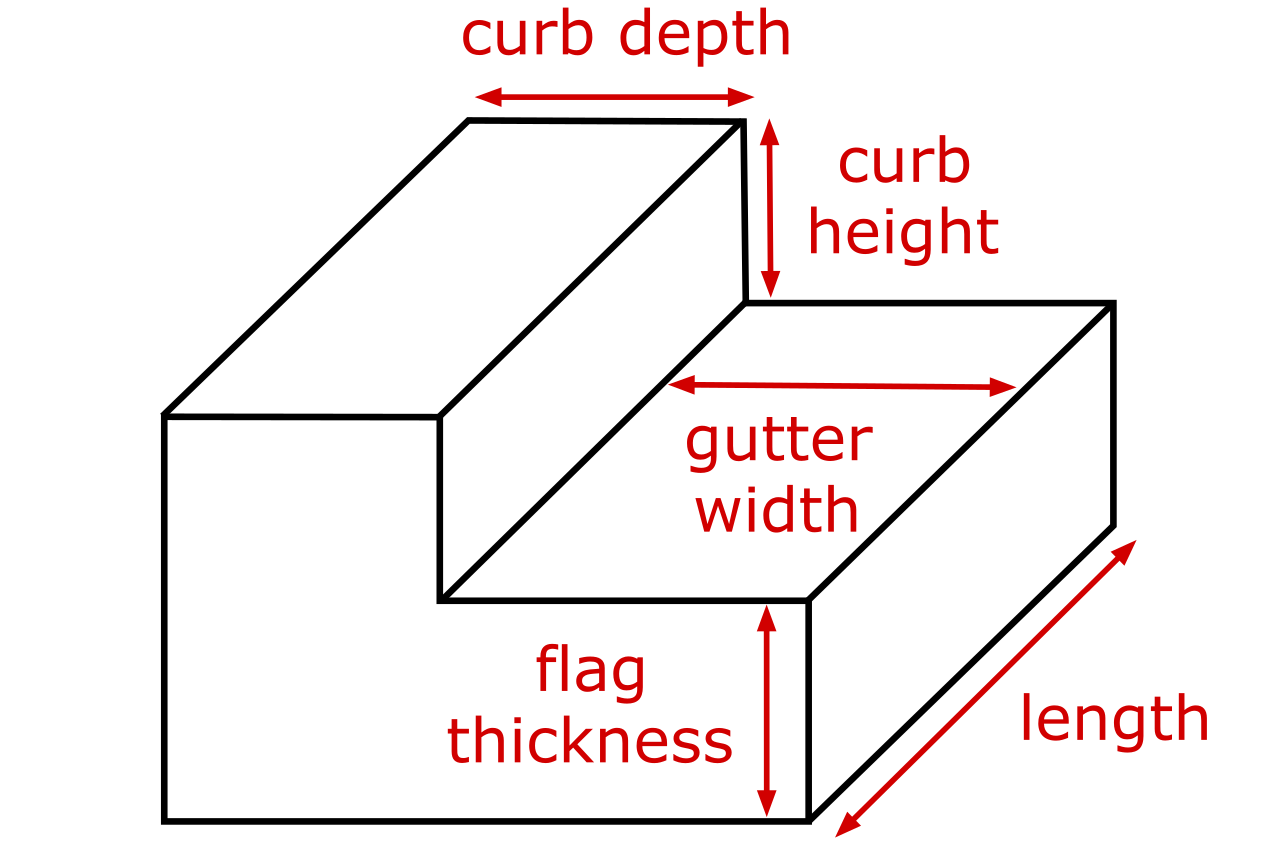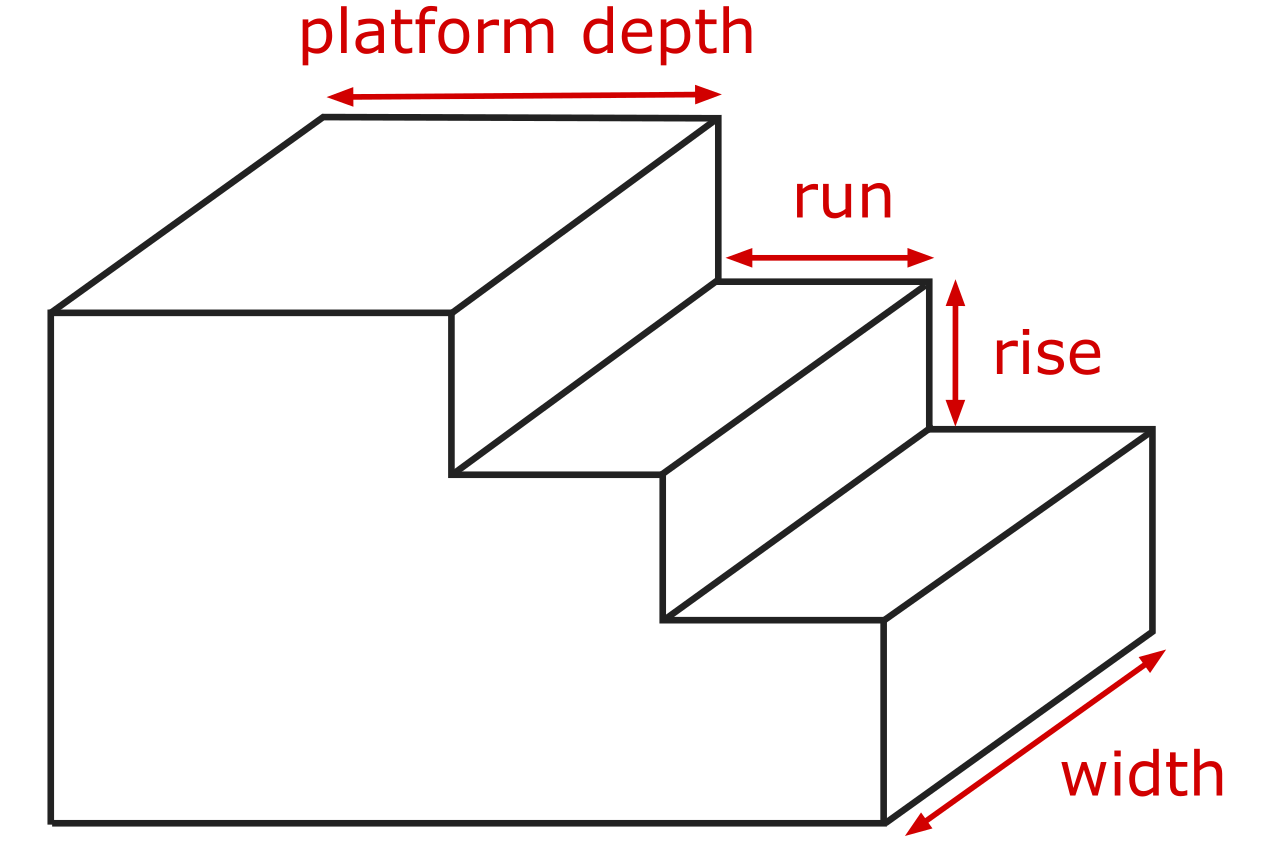Optionally enter the price per cubic yard
\$
Ready mix averages \$125 - \$165 per cubic yard

## Concrete Estimate:

 Cubic Yards: Cubic Meters:

### Pre-mixed Bags

 50-lb Bags: 60-lb Bags: 80-lb Bags:

### Estimated Concrete Cost

\$
Learn how we calculated this below

## How to Calculate Concrete for Footings

Many structures from porches and decks to homes and buildings use concrete footings for support. These footings may be small and meant to support a post or column, or they may be large and designed to carry the load of walls and roofs.

There are various types of footings that you can use to support a structure, and the type of footing will determine how to calculate the concrete needed to fill it. We’ll cover the calculations for a few types of footings below.

Pad footings are a type of isolated footing that spread a concentrated load from a pier or column. They can be rectangular or circular. These footings may be used for things like decks that need posts to support the structure above; the posts sit on the concrete footing.For a rectangular pad footing, you can calculate the volume of concrete needed for each one in cubic yards by multiplying the length L, width W, and depth D in feet, then dividing by 27.

cu yd = L(ft) × W(ft) × D(ft) / 27

For a circular pad footing, you can calculate the volume of concrete by multiplying the radius r squared in feet by the depth D in feet by pi, then dividing by 27.

The radius is half of the diameter, so if you are pouring a footing with a diameter of 4 feet, the radius is 2 feet. For smaller footings, you may want to make your calculations in inches. Rather than dividing by 27, you will need to divide by 46,660 to convert from inches to cubic yards.

cu yd = π × r(ft)² × D(ft) / 27

### Continuous/Strip Footings

Strip footings are a type of continuous footing that spread a concentrated load from a wall or multiple columns or piers. They are often used as the base of a foundation to provide a solid base and lateral support from shifting.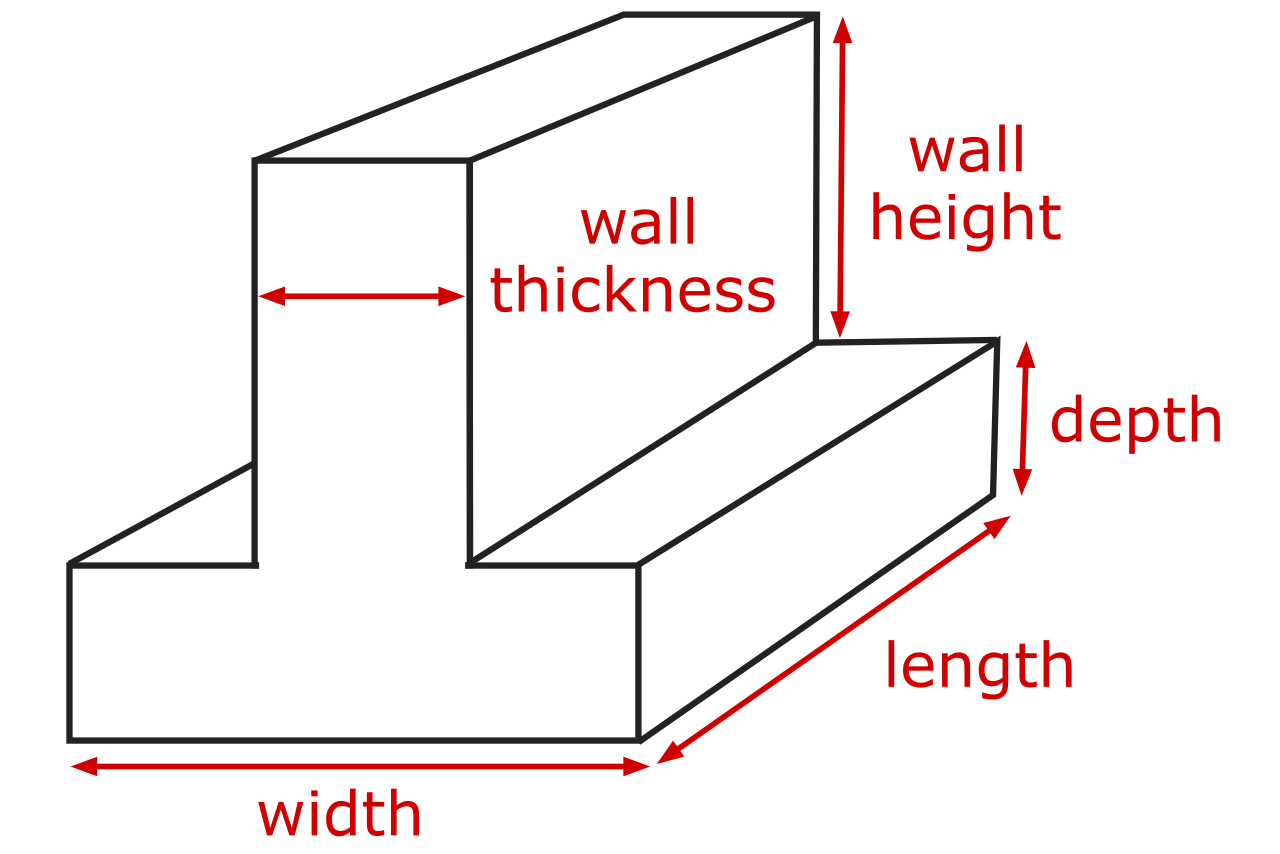To estimate the volume needed for a strip footing, measure the total linear footage of the footing, then multiply that by the width and depth in feet, then divide that by 27 to get the volume in cubic yards.

This is the same process as estimating a pad, but the length will generally be longer with a continuous footing.

In some cases, you can pour the foundation wall or a stem wall in a single, monolithic pour. When it’s an option, this can be advantageous to reduce pour time and curing time, and can consolidate two short loads into a single load.

To calculate the concrete needed for this type of pour, follow the steps above to estimate the volume of the base of the footing, then estimate the volume of the wall section. Then, add the volumes of each section together to get the total volume.

### Columns or Piers

Columns or piers are often used as the foundation for structures that need a foundation that is embedded deep into the soil. They’re also often used to support decks and porches on homes.You can estimate the volume of concrete needed for a column or pier, just like with a circular pad footing.

For a circular pad footing, you can calculate the volume of concrete by multiplying the radius r squared in feet by the depth D in feet by pi, then dividing by 27.

cu yd = π × r(ft)² × D(ft) / 27

Thus, the volume in cubic yards is equal to pi times the radius in feet squared, times the depth in feet, divided by 27.

In many cases, a pad or flared base is necessary to spread the concentrated load from the pier and prevent it from sinking.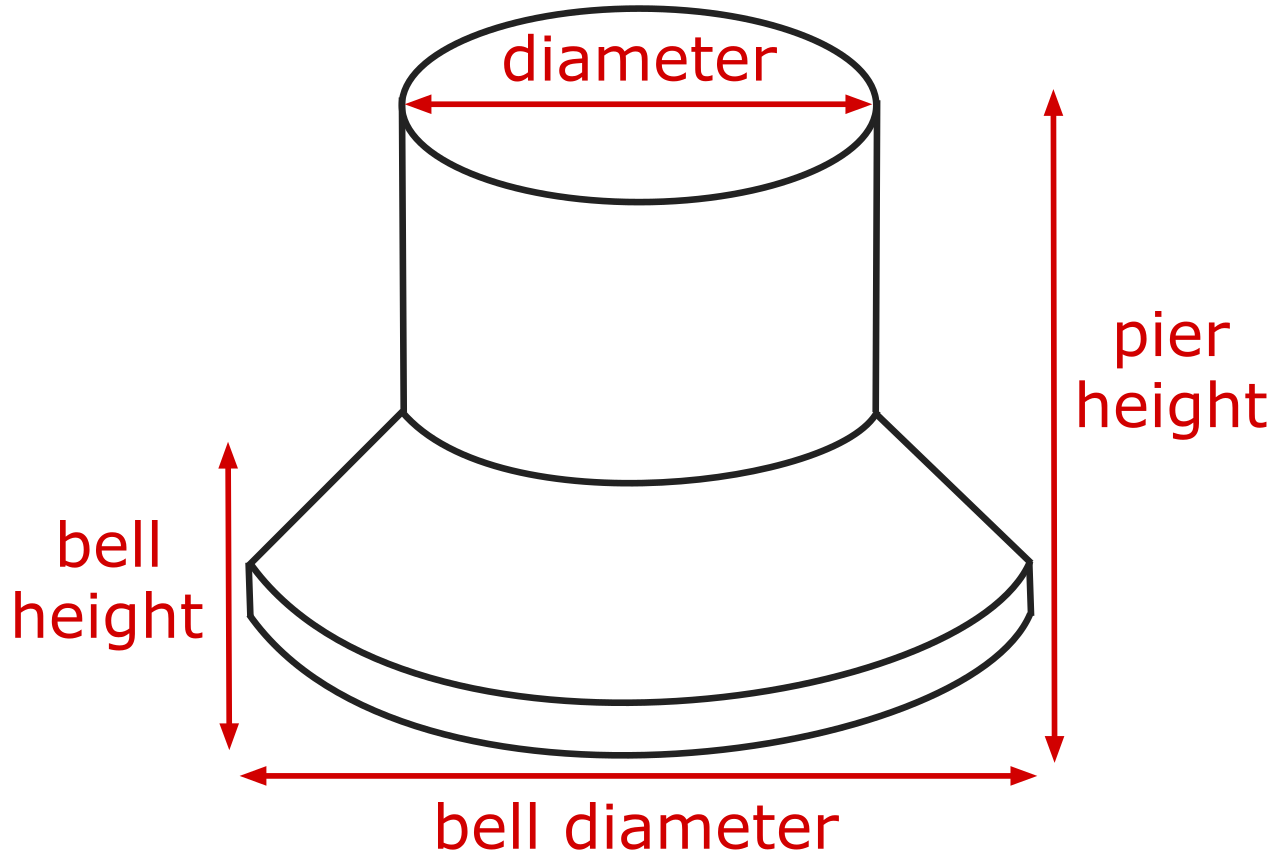If you’re using a form for this, the manufacturer often includes the volume needed to fill the bell footing on their spec sheet. Then add this to the volume needed to fill the column above it.

You can also calculate this yourself, if necessary, by using the formula for a conical frustum where the base radius is R, and the column radius is r.

volume = 1 / 3 π × D × (r² × rR × R²)

Keep in mind that you’ll need to convert this to cubic yards, then add it to the concrete needed for the upper portion of the column.

In the case of any of these foundations, we suggest ordering 10-15% additional material to account for spillage or forms that aren’t exactly to dimension. When digging, often the wall will have voids or may be slightly deeper in some places than others, which can result in short estimates.

If you’re thinking about hiring this project out, check out our guides on the cost to install footings, deck footings, or a foundation.